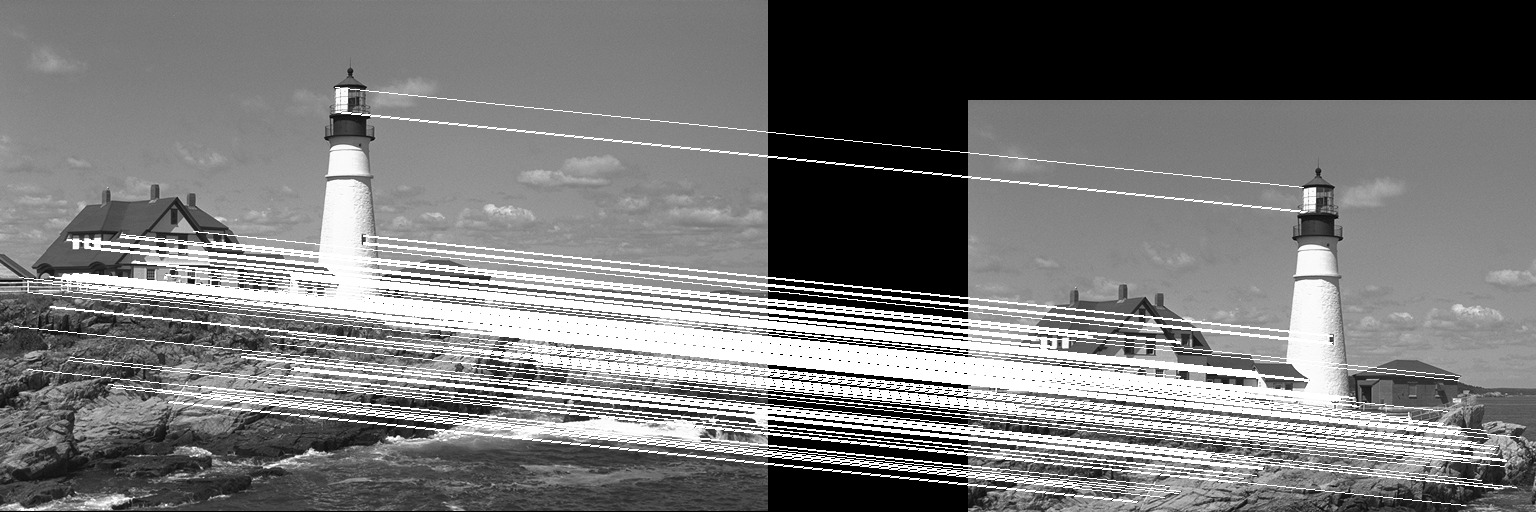BRIEF (Binary Robust Independent Elementary Features) is an efficient feature point descriptor. It is highly discriminative even when using relatively few bits and is computed using simple intensity difference tests. BRIEF does not have a sampling pattern thus pairs can be chosen at any point on the SxS patch.

To build a BRIEF descriptor of length n, we need to determine n pairs (Xi,Yi). Denote by X and Y the vectors of point Xi and Yi, respectively.

In ImageFeatures.jl we have five methods to determine the vectors X and Y :

• random_uniform : X and Y are randomly uniformly sampled
• gaussian : X and Y are randomly sampled using a Gaussian distribution, meaning that locations that are closer to the center of the patch are preferred
• gaussian_local : X and Y are randomly sampled using a Gaussian distribution where first X is sampled with a standard deviation of 0.04*S^2 and then the Yi’s are sampled using a Gaussian distribution – Each Yi is sampled with mean Xi and standard deviation of 0.01 * S^2
• random_coarse : X and Y are randomly sampled from discrete location of a coarse polar grid
• center_sample : For each i, Xi is (0, 0) and Yi takes all possible values on a coarse polar grid

As with all the binary descriptors, BRIEF’s distance measure is the number of different bits between two binary strings which can also be computed as the sum of the XOR operation between the strings.

BRIEF is a very simple feature descriptor and does not provide scale or rotation invariance (only translation invariance). To achieve those, see ORB, BRISK and FREAK.

## Example

Let us take a look at a simple example where the BRIEF descriptor is used to match two images where one has been translated by (100, 200) pixels. We will use the lena_gray image from the TestImages package for this example.

Now, let us create the two images we will match using BRIEF.

using ImageFeatures, TestImages, Images, ImageDraw, CoordinateTransformations

img = testimage("lighthouse")
img1 = Gray.(img)
trans = Translation(-100, -200)
img2 = warp(img1, trans, axes(img1))
 Downloading artifact: images

To calculate the descriptors, we first need to get the keypoints. For this tutorial, we will use the FAST corners to generate keypoints (see fastcorners).

keypoints_1 = Keypoints(fastcorners(img1, 12, 0.4))
keypoints_2 = Keypoints(fastcorners(img2, 12, 0.4))

To create the BRIEF descriptor, we first need to define the parameters by calling the BRIEF constructor.

brief_params = BRIEF(size = 256, window = 10, seed = 123)

Now pass the image with the keypoints and the parameters to the create_descriptor function.

desc_1, ret_keypoints_1 = create_descriptor(img1, keypoints_1, brief_params)
desc_2, ret_keypoints_2 = create_descriptor(img2, keypoints_2, brief_params)

The obtained descriptors can be used to find the matches between the two images using the match_keypoints function.

matches = match_keypoints(ret_keypoints_1, ret_keypoints_2, desc_1, desc_2, 0.1)

We can use the ImageDraw.jl package to view the results.

grid = hcat(img1, img2)
offset = CartesianIndex(0, size(img1, 2))
map(m -> draw!(grid, LineSegment(m, m + offset)), matches)